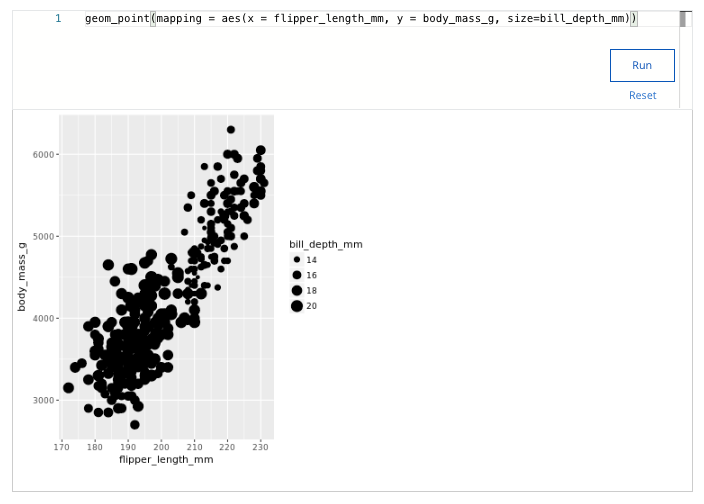## Week 4 – More about visualizations, aesthetics, and annotations – Shuffle Q/A 1

#### 13. Which code snippet will make all of the bars in the plot have different colors based on their heights?

• ggplot(data = buildings) +

geom_bar(mapping = aes(x = construction_year), color=height)

• ggplot(data = buildings) +

geom_bar(mapping = aes(x = construction_year)) +

color(“height”)

• ggplot(data = buildings) +

geom_bar(mapping = aes(x = construction_year, color=height))

• ggplot(data = buildings) +

geom_bar(mapping = aes(x = construction_year)) +

color(height)

#### 14. What is the purpose of the facet_wrap() function?

• Modify the visual characteristic of a data point
• Modify ggplot visuals to be three-dimensional
• Create text inside a plot area
• Create subplots in a grid of two variables

#### 15. A data analyst uses the annotate() function to create a text label for a plot. Which attributes of the text can the analyst change by adding code to the argument of the annotate() function? Select all that apply.

• Change the font style of the text.
• Change the color of the text.
• Change the size of the text.
• Change the text into a title for the plot.

#### 16. Which statement about the ggsave() function is correct?

• ggsave() exports the last plot displayed by default.
• ggsave() is run from the Plots Tab in RStudio.
• ggsave() is the only way to export a plot.
• ggsave() is unable to save .png files.

#### 17. Which of the following statements about ggplot is true?

• ggplot allows analysts to create plots using a single function.
• ggplot is the default plotting package in base R.
• ggplot allows analysts to create different types of plots.
• ggplot is designed to make cleaning data easy.

#### 18. A data analyst creates a plot using the following code chunk: ggplot(data = buildings) + geom_bar(mapping = aes(x = construction_year, color = height))Which of the following represents a variable in the code chunk?

• construction_year
• mapping
• data
• ggplot

#### 19. Which code snippet will make all of the bars in the plot purple?

• ggplot(data = buildings) +

geom_bar(mapping = aes(x = construction_year, color=”purple”))

• ggplot(data = buildings) +

geom_bar(mapping = aes(x = construction_year)) +

color(“purple”)

• ggplot(data = buildings) +

geom_bar(mapping = aes(x = construction_year, color=height))

• ggplot(data = buildings) +

geom_bar(mapping = aes(x = construction_year), color=”purple”)

#### 20. A data analyst is working with the following plot and gets an error caused by a bug. What is the cause of the bug?ggplot(data = penguins) %>% geom_point(mapping = aes(x = flipper_length_mm, y = body_mass_g))

• The code uses a pipe instead of a plus sign.
• A missing closing parenthesis needs to be added.
• The pipe should be at the beginning of the second line.
• A function name needs to be capitalized.

#### 21. You are working with the penguins dataset. You create a scatterplot with the following code chunk: ggplot(data = penguins) + geom_point(mapping = aes(x = flipper_length_mm, y = body_mass_g))You want to highlight the different penguin species in your plot. Add a code chunk to the second line of code to map the aesthetic size to the variable bill_depth_mm.NOTE: the three dots (...) indicate where to add the code chunk. You may need to scroll in order to find the dots.• 2 – 9
• 31 – 40
• 20 – 31
• 14 – 20

#### 22. A data analyst has a scatter plot with crowded points that make it hard to identify a trend. What geometry function can they add to their plot to clearly indicate the trend of the data?

• geom_alpha()
• geom_bar()
• geom_jitter()
• geom_smooth()

## Shuffle Q/A 2

• subtitle()
• title()
• labs()
• annotate()

#### 24. By default, what plot does the ggsave() function export?

• The plot define the plots.config file
• The last displayed plot
• The plot defined in the Plots Tab of R Studio
• The first plot displayed